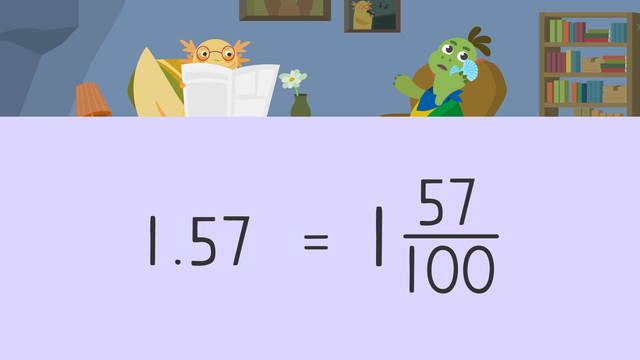# Decimals Greater than 1 as Fractions

Content Decimals Greater than 1 as FractionsRating

Ø 4.4 / 7 ratings
The authorsTeam Digital
Decimals Greater than 1 as Fractions
CCSS.MATH.CONTENT.4.NF.C.6

## Decimals Greater Than 1

When we have a number that is greater than 1 and written as a decimal, we can convert it so that it is written as a fraction. To write decimals greater than one as fractions, it is important to identify the place value for decimals greater than 1. This helps to know what the denominator is. Let’s look at the number one and fifty-seven hundredths as an example.## Decimals Greater Than 1 as Fractions

The first step is to set up a place value chart to the hundredths place, and write the number on the place value chart. This means one goes in the ones place, five goes in the tenths place, and seven goes in the hundredths place.Next, we write all the numbers as a fraction. One whole as a fraction is one over one. Five tenths as a fraction is five over ten, and seven hundredths as a fraction is seven over one hundred.Then, we need to convert the fractions to have common, or the same, denominators. In this example, we will convert all fractions to have the denominator one hundred. We multiply the numerator and denominator by one hundred for one over one to get one hundred over one hundred. Then, we multiply the numerator and denominator by ten for five over ten to get fifty over one hundred. Seven over one hundred does not need converting.Now, we add all the fractions together. The denominator remains one hundred, and we add one hundred plus fifty plus seven to give us one hundred and fifty-seven for the numerator.Since one hundred and fifty-seven is greater than one hundred, it is an improper fraction. We can rewrite this as the mixed fraction, one and fifty-seven over one hundred.This means that one and fifty-seven hundredths as a fraction is one and fifty-seven over one hundred.## Decimals Greater Than 1 – Summary

Decimals greater than 1 are numbers greater than one with a decimal place. You can display decimals greater than 1 as fractions or as mixed numbers. When you need to represent numbers with decimals, you can use a place value chart to the hundredths place or multiply the number by 100. Follow these steps to identify the place value of decimals:

Step # What to do
1 Set up a place value chart to the hundredths
place and write down the number.
2 Write all the numbers as a fraction.
One whole is one over one as a fraction.
3 Convert the fractions to have common